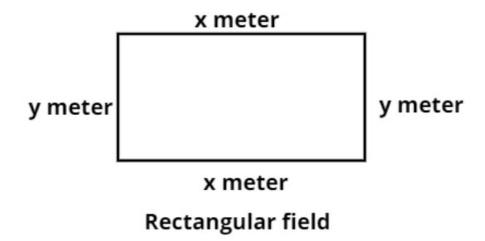QuestionAnswers

# A rectangular field has its length and breadth in the ratio 5 : 3. Its area is 3.75 hectares. Find the cost of the fencing if it is at Rs. 5 per meter.$\left( A \right)$ Rs. 400$\left( B \right)$ Rs. 4000$\left( C \right)$ Rs. 1000$\left( D \right)$ Rs. 18,000Hint – In this question assume any different variables be the length and the breadth of the rectangular field and calculate length in terms of breadth or vice versa as ratio is given and use the concept that area of the rectangular field is length times breadth so use these concepts to reach the solution of the question.Given data:
Ratio of length and breadth of the rectangular field is (5:3)............. (1)
Its area (A) is = 3.75 hectares................... (2)
Now let the length of the rectangular field be x meter and breadth of the rectangular field is y meter.
So from equation (1) we have,
$\Rightarrow \dfrac{x}{y} = \dfrac{5}{3}$
$\Rightarrow x = \dfrac{5}{3}y$.................. (3)
Now as we know that the area of the rectangle is the product of the length and the breadth of the rectangle.
So the area of the rectangle is
$\Rightarrow A = xy$Square meter.
Now from equation (3) we have,
$\Rightarrow A = \dfrac{5}{3}y\left( y \right) = \dfrac{5}{3}{y^2}$Square meter.
Now from equation (2) we have,
$\Rightarrow$A = 3.75 hectare$= \dfrac{5}{3}{y^2}$Square meter................ (4)
Now as we know that 1 hectare = 10000 square meter.......... (5)
Therefore from equation (4) and (5) we have,
$\Rightarrow$A = 3.75 $\times$ 10000 square meter$= \dfrac{5}{3}{y^2}$Square meter
Now simplify this equation we have,
$\Rightarrow 37500 = \dfrac{5}{3}{y^2}$
Now calculate the value of y (i.e. breadth) we have
$\Rightarrow y = \sqrt {\dfrac{{37500\left( 3 \right)}}{5}} = \sqrt {22500} = 150$ Meter.
Now from equation (3) we have,
$\Rightarrow x = \dfrac{5}{3}\left( {150} \right) = 250$ Meter.
Now the cost of fencing is the perimeter of the rectangle.
As we know that the perimeter of any shape is the sum of all the side lengths of the shape.
So the perimeter of the rectangular field = 2(x + y)
Now substitute the values we have,
So the perimeter or the fencing required for the rectangular field = 2(250 + 150) = 2(400) = 800 meter.
Now it is given that the cost of fencing is at Rs. 5 per meter.
So the cost of 800 m fencing is = 800(5) = Rs. 4000
So this is the required answer.
Note – Whenever we face such types of questions the key concept we have to remember is that always recall that the perimeter of any shape is the sum of all the side lengths of the shape, so find out the length and breadth of the rectangular field then substitute these values in the formula of the perimeter as above and simplify we will get the required answer.
View Notes
Electric Field of a SphereMotion of a Charged Particle in Magnetic FieldDifference Between Electric Field and Magnetic FieldRelation Between the Length of a Given Wire and Tension for Constant Frequency Using SonometerArea of a Sector of a Circle FormulaArea of a Rhombus FormulaCBSE Class 7 Maths Chapter 6 - Triangle and Its Properties FormulasBiotechnology and its ApplicationsType of Protein and Its FunctionsPreparation of Hydrogen and Its UsesImportant Questions for CBSE Class 6 English A Pact with The Sun Chapter 8 - A Pact with the SunImportant Questions for CBSE Class 7 Social Science - Social and Political Life Chapter 8 - A Shirt In The MarketImportant Questions for CBSE Class 8 English Honeydew Chapter 8 - A Short Monsoon DiaryImportant Questions for CBSE Class 6 English Honeysuckle Chapter 8 - A Game of ChanceImportant Questions for CBSE Class 8 Social Science Our Past 3 Chapter 4 - Tribals, Dikus and the Vision of a Golden AgeImportant Questions for CBSE Class 8 English Honeydew Chapter 7 - A Visit to CambridgeImportant Questions for CBSE Class 8 Social Science - Social and Political Life Chapter 8 - Confronting MarginalisationImportant Questions for CBSE Class 6 English A Pact with The Sun Chapter 1 - A Tale of Two BirdsCBSE Class 7 Science Electric Current and Its Effects WorksheetsImportant Questions for CBSE Class 8 Maths Chapter 8 - Comparing QuantitiesCBSE Class 10 Hindi A Question Paper 2020Hindi A Class 10 CBSE Question Paper 2009Hindi A Class 10 CBSE Question Paper 2015Hindi A Class 10 CBSE Question Paper 2016Hindi A Class 10 CBSE Question Paper 2012Hindi A Class 10 CBSE Question Paper 2010Hindi A Class 10 CBSE Question Paper 2007Hindi A Class 10 CBSE Question Paper 2013Hindi A Class 10 CBSE Question Paper 2008Hindi A Class 10 CBSE Question Paper 2014RS Aggarwal Class 8 Mathematics Solutions for Chapter-18 Area of a Trapezium and a PolygonRS Aggarwal Solutions Class 8 Chapter-18 Area of a Trapezium and a Polygon (Ex 18C) Exercise 18.3RS Aggarwal Solutions Class 8 Chapter-18 Area of a Trapezium and a Polygon (Ex 18B) Exercise 18.2RS Aggarwal Solutions Class 8 Chapter-18 Area of a Trapezium and a Polygon (Ex 18A) Exercise 18.1HC Verma Class 12 Physics Part-2 Solutions for Chapter 29 - Electric Field and PotentialRD Sharma Solutions for Class 9 Maths Chapter 21 - Surface Area and Volume of a SphereRD Sharma Solutions for Class 9 Maths Chapter 18 - Surface Area and Volume of a Cuboid and CubeNCERT Solutions for Class 8 Maths Chapter 8 Comparing Quantities in HindiNCERT Solutions for Class 7 Maths Chapter 6 The Triangle and Its PropertiesRS Aggarwal Solutions Class 8 Chapter-8 Linear Equations (Ex 8B) Exercise 8.2